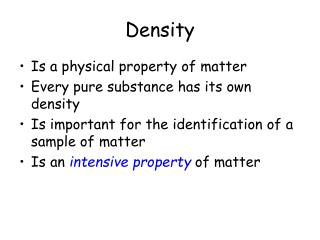DownloadDownload PresentationDensity

# Density

Télécharger la présentation## Density

- - - - - - - - - - - - - - - - - - - - - - - - - - - E N D - - - - - - - - - - - - - - - - - - - - - - - - - - -
##### Presentation Transcript

1. Density • Is a physical property of matter • Every pure substance has its own density • Is important for the identification of a sample of matter • Is an intensive property of matter

2. Density • Density is defined as mass per unit volume. • The relationship can be written mathematically as: OR • units: solids [ g / cm3 ] • liquids[ g / mL] • gases [ g / L]

3. Comparison of Densities Each cube represents a volume of 1.00 cm3. The mass of each sample increases while the volume remains constant.

4. Example: • The sample of matter you have has a mass of 3.51 g and occupies a volume of 1.30 cm3.

5. EXAMPLE: You need to add 45.0 g of alcohol to a sample. It is difficult to weigh an exact mass of a liquid. It is much easier to measure an exact volume. Using the density of the alcohol (0.789 g/cm3), you can determine what volume you would need to add to your sample so that you would be adding only the 45.0 grams. = 57.0 mL in the correct number of sig figs

6. Determining the Volume • In order to figure the density we need to determine the volume: • For regularly shaped objects we use direct measurement:

7. Used for irregular shaped objects Select a graduated cylinder large enough to put the object in Add water and accurately record the initial volume. Measuring Volume by Displacement

8. SLOWLY add the object to be measured: rubber stopper rock metal cylinder Read the new level of the water The water will rise an amount equal to the volume of the object

9. What not to do

10. Here’s what the cylinder should look like

11. Do the math 1 . Original reading of volume • Final volume of water • This will equal the volume of the object • 22.5 mL - 17.4 mL =  5.1 mL •     Therefore, the volume of the object by displacement is 5.1 mL

12. EXAMPLE: An irregularly shaped piece of quartz was place in a graduated cylinder containing 20.45 mL of water. The volume of the ater rose to 24.35 mL. What is the volume of the water?

13. Classroom Exercises: Blue Block • Mass = 79.4 grams • Volume=29.8 cubic cm. • D = 79.4 g ÷ 29.8 cm3 = 2.66443 = 2.66 g/ cm3

14. Green Block • Mass= 25.4 grams • Volume=29.8 cubic cm • D = 25.4 g ÷ 29.8 cm3 = .85235 = .852 g/ cm3 Which block is more dense?

15. Cylinder Radius = 3.5cm Length = 7.25 cm Mass = 32.3 g What is the volume? V = π X radius2 X height π = 3.14 What is the density?

16. Sphere • Diameter = 7.0cm • Radius = 3.5cm • Mass = 32.3 g • What is the volume? V = 4/3 π X radius3 π = 3.14 What is the density?

17. Density • Can also be used as a means of separating components of matter • Ex: if 2 solids of a different density are mixed together, the solid with the greater density will gravitate to the bottom • When a solid object is placed in a liquid that has a great density, the solid object will float. • We will test this hypothesis in lab.